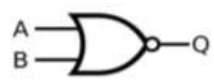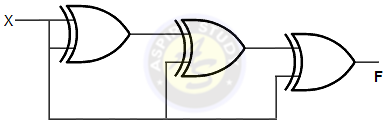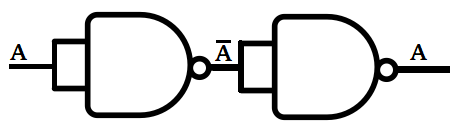## Aspire's Library

A Place for Latest Exam wise Questions, Videos, Previous Year Papers,
Study Stuff for MCA Examinations

## NIMCET Previous Year Questions (PYQs)

#### NIMCET Computer PYQ

NIMCET PYQ
If we can generate a maximum of 4 Boolean functions using n Boolean variables, what will be minimum value of n?

NIMCET PREVIOUS YEAR QUESTION

NIMCET Computer PYQNIMCET Boolean algebra PYQ

#### Solution

NIMCET PYQ
The Process when processor fetch or decode another instruction during the execution of current instruction is called

NIMCET Previous Year PYQNIMCET NIMCET 2021 PYQ

#### Solution

Pipelining is the process of accumulating instruction from the processor through a pipeline. It allows storing and executing instructions in an orderly process. It is also known as pipeline processing. Pipelining is a technique where multiple instructions are overlapped during execution.

NIMCET PYQ
Which of the following is used by ALU to store the intermediate results?

NIMCET Previous Year PYQNIMCET NIMCET 2021 PYQ

#### Solution

An accumulator is a type of register included in a CPU. It acts as a temporary storage location which holds an intermediate value in mathematical and logical calculations. Intermediate results of an operation are progressively written to the accumulator, overwriting the previous value. For example, in the operation "3 + 4 + 5," the accumulator would hold the value 3, then the value 7, then the value 12. The benefit of an accumulator is that it does not need to be explicitly referenced, which conserves data in the operation statement.

NIMCET PYQ
One TeraByte(TB)=_________GB and One ExaByte(EB)=_______GB

NIMCET Previous Year PYQNIMCET NIMCET 2021 PYQ

#### Solution

UnitShortenedCapacity
Bitb1 or 0 (on or off)
ByteB8 bits
KilobyteKB1024 bytes
MegabyteMB1024 kilobytes
GigabyteGB1024 megabytes
TerabyteTB1024 gigabytes
PetabytePB1024 terabytes
ExabyteEB1024 petabytes
ZettabyteZB1024 exabytes
YottabyteYB1024 zettabytes

NIMCET PYQ
The Cache Memory is  more effective because of

NIMCET Previous Year PYQNIMCET NIMCET 2021 PYQ

#### Solution

Locality of reference refers to a phenomenon in which a computer program tends to access same set of memory locations for a particular time period. In other words, Locality of Reference refers to the tendency of the computer program to access instructions whose addresses are near one another.

NIMCET PYQ
Which of the following is the fastest means of memory access for CPU?

NIMCET Previous Year PYQNIMCET NIMCET 2021 PYQ

#### Solution

Registers are a type of computer memory used to quickly accept, store, and transfer data and instructions that are being used immediately by the CPU. The registers used by the CPU are often termed as Processor registers.

NIMCET PYQ
The number (2217)8 is equivalent to

NIMCET Previous Year PYQNIMCET NIMCET 2021 PYQ

#### Solution

(2217)8 =(010010001111)8
Pair of 4 Bits
1111= F
1000=8
0100=4
(010010001111)=(48F)16

NIMCET PYQ
To fetch data from secondary memory which one of the following register is used

NIMCET Previous Year PYQNIMCET NIMCET 2021 PYQ

#### Solution

MAR register is used to access data and instructions from memory during the execution phase of instruction. MAR holds the memory location of data that needs to be accessed. When reading from memory, data addressed by MAR is fed into the MDR (memory data register) and then used by the CPU. When writing to memory, the CPU writes data from MDR to the memory location whose address is stored in MAR. MAR, which is found inside the CPU, goes either to the RAM (random-access memory) or cache.

NIMCET PYQ
The binary multiplication 00*11 will give

NIMCET Previous Year PYQNIMCET NIMCET 2021 PYQ

#### Solution

0*3=0

NIMCET PYQ
Consider a computer system with speed of 106  instructions per second. A program P, having 2n2  steps is run on this system, where n is the input size. If n = 10000, what is the execution time for P?

NIMCET Previous Year PYQNIMCET NIMCET 2021 PYQ

#### Solution

$\text{Speed of computer} = 10^6 \text{per second}$
$\text{For n} =10000=10^4$
$$Time = \frac{\text{No of tasks}}{\text{Speed of computer}}$$
$$Time = \frac{2n^2}{10^6}$$
$$= \frac{2\times (10^4)^2}{10^6}$$
$$= \frac{2\times 10^8}{10^6}$$
$$=2 \times 10^2$$
$$= 200sec$$

NIMCET PYQ
To access the I/O devices the status flags is continuously checked in

NIMCET Previous Year PYQNIMCET NIMCET 2021 PYQ

#### Solution

Programmed I/O: In program-controlled I/O, the processor program controls the complete data transfer. So only when an I/O transfer instruction is executed, the transfer could take place. It is required to check that device is ready/not for the data transfer in most cases.  Usually, the transfer is to & from a CPU register & peripheral. Here, CPU constantly monitors the peripheral. Here, until the I/O unit indicates that it is ready for transfer, the CPU wait & stays in a loop. It is time-consuming as it keeps the CPU busy needlessly.

NIMCET PYQ
Which one of the following Boolean algebraic rule is correct?

NIMCET Previous Year PYQNIMCET NIMCET 2017 PYQ

#### Solution

A + A'B = (A + A') . (A + B)
=  1 . (A + B)
= A + B

NIMCET PYQ
The representation of a floating point binary number +1001.11 in 8 bit fraction and 6 bit exponent format is

NIMCET Previous Year PYQNIMCET NIMCET 2017 PYQ

#### Solution

NIMCET PYQ
Which term is redundant in the expression AB + A'C + BC ?

NIMCET Previous Year PYQNIMCET NIMCET 2017 PYQ

#### Solution

NIMCET PYQ
Let the memory access time is 10 miliseconds and cache hit ratio 15%. The effective memory access time is

NIMCET Previous Year PYQNIMCET NIMCET 2017 PYQ

#### Solution

NIMCET PYQ
Which of the following is the representation of decimal number (- 147) in 2's compliment notation on a 12-bit machine?

NIMCET Previous Year PYQNIMCET NIMCET 2017 PYQ

#### Solution

NIMCET PYQ
The first instruction of bootstrap loader program of an operating system is stored in

NIMCET Previous Year PYQNIMCET NIMCET 2017 PYQ

#### Solution

NIMCET PYQ
Consider the equation (40)x = (132)y is some bases x and y. Then a possible set of value of x and y are

NIMCET Previous Year PYQNIMCET NIMCET 2017 PYQ

#### Solution

$\begin{array}{ll}{{{(40})}_x={{(132)}}_y} \\ {\Rightarrow4\times{x}^1}+0\times{x}^0=1\times{y}^2+3\times{y}^1+2\times{y}^0 \\ {\Rightarrow4x+0={y}^2+3y^{}+2} \\ {4x={y}^2+3y^{}+2}\end{array}$

NIMCET PYQ
The smallest integer that can be represented by an 8 bit number in 2's complement form is

NIMCET Previous Year PYQNIMCET NIMCET 2017 PYQ

#### Solution

NIMCET PYQ
Which of the following in a functionally complete set of gates?
I. NAND                  II. NOR

NIMCET Previous Year PYQNIMCET NIMCET 2017 PYQ

#### Solution

NIMCET PYQ
The total number binary function that can be defined using n Boolean variables is

NIMCET Previous Year PYQNIMCET NIMCET 2017 PYQ

#### Solution

NIMCET PYQ
Assume x' represents negation of x the Boolean function x'y' + xy + x'y is equivalent to?

NIMCET Previous Year PYQNIMCET NIMCET 2020 PYQ

#### Solution

NIMCET PYQ
The memory unit which directly communicates with the CPU is known as

NIMCET Previous Year PYQNIMCET NIMCET 2020 PYQ

#### Solution

NIMCET PYQ
Dynamic RAM consumes……. Power and ……than Static RAM

NIMCET Previous Year PYQNIMCET NIMCET 2020 PYQ

#### Solution

NIMCET PYQ
The binary equivalent of (234.125)10?

NIMCET Previous Year PYQNIMCET NIMCET 2020 PYQ

#### Solution

NIMCET PYQ
Determine the octal equivalent of (432267)10?

NIMCET Previous Year PYQNIMCET NIMCET 2020 PYQ

#### Solution

NIMCET PYQ
One Exabyte is equal to …

NIMCET Previous Year PYQNIMCET NIMCET 2020 PYQ

#### Solution

NIMCET PYQ
Consider the following circuit.How many minimum numbers of two input NAND gates are required to design the above circuit?

NIMCET Previous Year PYQNIMCET NIMCET 2020 PYQ

#### Solution

NIMCET PYQ
The time required for fetching and execution of one simple machine instruction is known as

NIMCET Previous Year PYQNIMCET NIMCET 2020 PYQ

#### Solution

NIMCET PYQ
The equivalence of given expression x+x'y with Boolean theorem is….

NIMCET Previous Year PYQNIMCET NIMCET 2020 PYQ

#### Solution

x+x'y
=(x+x')(x+y)
=(x+y)

NIMCET PYQ
The logic XOR operation of (4AC0)16 and (B53F)16 results

NIMCET Previous Year PYQNIMCET NIMCET 2020 PYQ

#### Solution

NIMCET PYQ
In IEEE single precision floating point representation, exponent is represented in ______

NIMCET Previous Year PYQNIMCET NIMCET 2019 PYQ

#### Solution

NIMCET PYQ
With 4-bit 2's complement arithmetic, which of the following addition will result in overflow?

NIMCET Previous Year PYQNIMCET NIMCET 2019 PYQ

#### Solution

NIMCET PYQ
If the 2's complement representation of a number is (011010)2 , what is its equivalent hexadecimal representation?

NIMCET Previous Year PYQNIMCET NIMCET 2019 PYQ

#### Solution

NIMCET PYQ
For the circuit shown below, the complement of the output F is _________NIMCET Previous Year PYQNIMCET NIMCET 2019 PYQ

#### Solution

NIMCET PYQ
If N is a 16-bit signed integer, then 2's complement representation of N is (F87B)16. The 2's complement representation of 8*N is

NIMCET Previous Year PYQNIMCET NIMCET 2019 PYQ

#### Solution

NIMCET PYQ
The base ( or radix) of the number system such that the following equation holds 312/20 = 131.1 is

NIMCET Previous Year PYQNIMCET NIMCET 2019 PYQ

#### Solution

NIMCET PYQ
Which of the following represents (D4)16 ?

NIMCET Previous Year PYQNIMCET NIMCET 2019 PYQ

#### Solution

NIMCET PYQ
How many Boolean expressions can be be formed with 3 Boolean variables?

NIMCET Previous Year PYQNIMCET NIMCET 2019 PYQ

#### Solution

NIMCET PYQ
In an 8 bit representation of computer system the decimal number 47 has to be subtracted from 38 and the result in binary 2's complement is _________

NIMCET Previous Year PYQNIMCET NIMCET 2019 PYQ

#### Solution

NIMCET PYQ
The maximum and minimum value represented in signed 16 bit 2's complement representations are

NIMCET Previous Year PYQNIMCET NIMCET 2022 PYQ

#### Solution

Range of 2's complement $-2^{n-1}$ to $2^{n-1}+1$

Range for 16 bits = $-2^{16-1}$ to $2^{16-1}+1$

Range for 16 bits = $-2^{15}$ to $2^{25}+1$

Range for 16 bits = $-32768$ to $32767$

NIMCET PYQ
The minimum number of NAND gates required for implementing the Boolean expression $AB+A\, \overline{B}C+A\, \overline{B}\, \overline{C}$

NIMCET Previous Year PYQNIMCET NIMCET 2022 PYQ

#### Solution

$AB+A\, \overline{B}C+A\, \overline{B}\, \overline{C}$
=$AB+AB'C+AB'C'$
=$AB+AB'(C+C')$
=$AB+AB'$
=$A(B+B')$
=ANIMCET PYQ
Which of the following is equivalent to the Boolean expression:
$(X+Y).(X+\overline{Y}).(\overline{X}+Y)$

NIMCET Previous Year PYQNIMCET NIMCET 2022 PYQ

#### Solution

$(X+Y).(X+\overline{Y}).(\overline{X}+Y)$
=$(X+Y)(X+Y')(X'+Y)$
=$(XX+XY+YX+YY')(X'+Y)$
=$(X+XY)(X'+Y)$
=$X(1+Y)(X'+Y)$
=$X(X'+Y)$
=$XX'+XY$
=$XY$

NIMCET PYQ
Suppose the largest n bit number requires ‘d’ digits in decimal representation. Which of the following relations between ‘n’ and ‘d’ is approximately correct

NIMCET Previous Year PYQNIMCET NIMCET 2022 PYQ

#### Solution

n bits binary number required d -decimal digits.

So, ${10}^d{\gt}{2}^n$

Take on both side

$\log _{10}({10}^d)\gt{\log _{10}({2}^n)}^{}$

$d{\gt}n\log _{10}(2)$

NIMCET PYQ
If a processor clock is rated as 2500 million cycles per second, then its clock period is:

NIMCET Previous Year PYQNIMCET NIMCET 2022 PYQ

#### Solution

we know that Frequency is defined as the number of cycles in one second

Number of cycle in 1 sec = 2500 million

=> Frequency = 2500 Mhz

we know that time period is the inverse of frequency and is defined as the time taken by one cycle.

$T = \frac{1}{F}$

$T = \frac{1}{2500 \times 10^{-6}}$

$T=4 \times 10^{-10}$ sec

NIMCET PYQ
Write the simplified form of the Boolean expression

NIMCET Previous Year PYQNIMCET NIMCET 2022 PYQ

#### Solution

=(A+C)A(D+D')+C(A+1)
=(A+C)+C
=A+C

NIMCET PYQ
FFFF will be the last memory location in a memory of size

NIMCET Previous Year PYQNIMCET NIMCET 2022 PYQ

#### Solution

The Hexadecimal digits are 0-9 and A-F. The Hexadecimal system represents numbers in16 symbols, zero to nine and ten to fifteen is represented by the English alphabet A-F.

The Hexadecimal character represents 4 bits.
The last memory location in a memory of size 64K is FFFF.

64K is $2^{16}$ bytes, i.e.
$16^4$ bytes = 1000 bytes in hexadecimal code.

The last accessible address is 1000-1 = FFFF.

NIMCET PYQ
‘Floating point representation' is used to represent

NIMCET Previous Year PYQNIMCET NIMCET 2022 PYQ

#### Solution

NIMCET PYQ
The Boolean expression AB+ AB' + A'C + AC is unaffected by the value of the Boolean variable

NIMCET Previous Year PYQNIMCET NIMCET 2022 PYQ

#### Solution

$\begin{array}{ll}{A(B+\overline{B})+C(A+\overline{A})} \\ {A+C}\, \end{array}$

NIMCET PYQ
If a signal passing through a gate is inhibited by sending a low into one of the inputs, and the output is HIGH, the gate is a(n):

NIMCET Previous Year PYQNIMCET NIMCET 2022 PYQ

#### Solution

Output is high if any of the input is low. The truth table for NAND gate is:
 A B Output 0 0 0 0 1 1 1 0 1 1 1 1
Table representing NAND Gate.

#### NIMCET

Online Test Series,
and More.

#### NIMCET

Online Test Series,